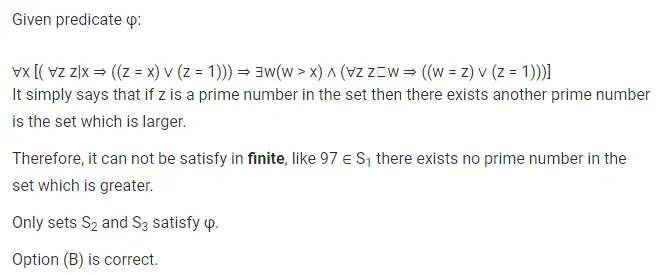# Gate CS-2019 Question Paper With Solutions

Q. 44 Consider the first order predicate formula:

`∀x [( ∀z z|x ⇒ ((z = x) ∨ (z = 1))) ⇒ ∃w(w > x) ∧ (∀z z⏐w ⇒ ((w = z) ∨ (z = 1)))]`

Here ‘a⏐b’ denotes that ‘a divides b’, where a and b are integers. Consider the following sets:

• S1: {1, 2, 3, …, 100}
• S2: Set of all positive integers
• S3: Set of all integers

Which of the above sets satisfy φ ?

(A) S1 and S3

(B) S2 and S3

(C) S1, S2 and S3

(D) S1 and S2